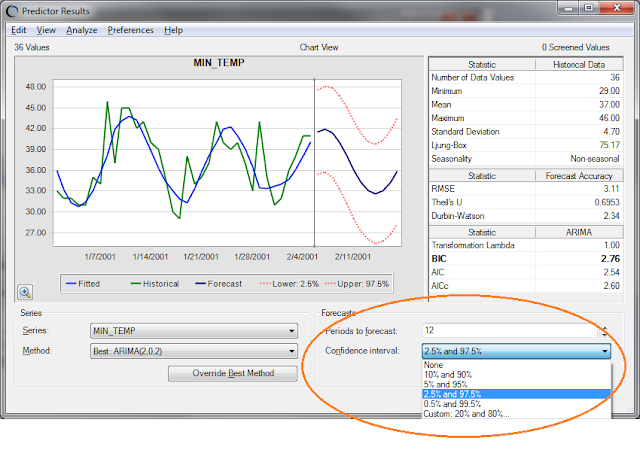Monday, March 5, 2012

Confidence Intervals (Prediction Intervals) in CB Predictor (Part 1): The Calculations

In the CB Predictor result window, we show the confidence interval (CI) [also known in the literature as Prediction Intervals (* See Below)] of the forecasts. Prof. Chatfield has a great paper about prediction intervals, their importance, and how to compute them - interested readers, please have a look.

The default CI’s in CB Predictor result window are at 2.5% and 97.5%, but this can be easily changed using the dropdown at the bottom-right corner of the result window (see figure 1). One can even use custom percentiles for the CI’s to show up in the result window (and also in the Predictor reports). Although this part is quite straight forward, there are quite a few steps in calculating the standard error which might be able to use some further explanation. In this and subsequent posts, we will discuss about some of the interesting stuff around the CI in Predictor.Figure 1: Result window

Confidence intervals for classic methods
The way we calculate the confidence intervals for classic forecasting methods (four non-seasonal and four seasonal methods) is not very obvious from our charts and reports. We use an empirical formula to calculate the confidence intervals for the forecast of each period.

Reference
The formula is from the following reference:
Bowerman, B.L., and R.T. O'Connell (contributor). Forecasting and Time Series: An Applied Approach (The Duxbury Advanced Series in Statistics and Decision Sciences). Belmont, CA: Duxbury Press. 1993.
See Section 8.6, Prediction Intervals (page 427).

Google Books project seem to have only snippets available from the book due to licensing issues, so if anyone is interested in the actual text and do not have access to the book, please feel free to contact me (or Oracle support, for that matter) to receive a scan of the relevant pages.

Assumptions
The method makes a few assumptions:
• Historical data amount is sufficiently large
• The forecast errors are normally distributed
Method summary
Without reproducing the formula for this calculation, which is already available in our reference manual and in the reference above, let us describe the process here. Note that, the symbols used in the formula in our manual (or in the book) are not the same as the symbols used in the description below, but the idea is the same.

Let's say we have data points for periods 1 to t: Y(1) to Y(t). We calculate the forecast for the period (t+1)-th using whichever forecasting method is selected. Let's say the forecast is F(t+1). From the fitted data (using the forecasting model) we also can calculate the RMSE, let’s assume that is RMSE(t+1). Now, the normal distribution assumption is defined as: N(F(t+1), RMSE(t+1)). Of course, this normal distribution is then used to calculate the CI’s at different percentile levels. Note here, that the fitted values which we get from the model, which are used to calculate both F(t+1) and RMSE(t+1), are nothing but 1-period ahead forecasts at each period, starting from period, say, k (*), and ending at period t, using the equations of the forecasting model. It is, as if, we were forecasting 1-period ahead at every period in (k,...,t).

(*) Important note: When I say the phrase “starting at period k”, k is the starting period where we can begin to calculate the 1-period ahead forecasts. For some models (like Double Moving Average, or the seasonal models), one can’t really start calculating 1-period ahead forecasts at period 2.

This is the insight that is used in the heuristic. For period (t+2), the forecast F(t+2) is obtained from the method equations. For the standard error, we calculate 2-period ahead forecasts at each period, starting from period, say, k+1, and ending at period t, and then calculate the RMSE of these values w.r.t. the dataset, calling it RMSE(t+2). The normal distribution assumption then, of course, is N(F(t+2), RMSE(t+2)), which is used in calculating CI’s. For period (t+3), we use the same procedure, except now we look at 3-period ahead forecasts, and so on.

The procedure is simple and intuitive, and works pretty well in most situations. Using the above description, one should be able to easily validate the numbers seen in Predictor by implementing a forecasting method and the standard error for that method in Excel.

Confidence Intervals for ARIMA methods
For Box-Jenkins ARIMA methods, we do not use the heuristic mentioned above. Rather, we use the theoretical formula to calculate the standard errors.

Reference
A reference for this procedure is below.
Box, G. E. P., Jenkins, G. M., and Reinsel, G. C. Time Series Analysis: Forecasting and Control. 4th ed. Hoboken, NJ: John Wiley & Sons. 2008. Chapter 5, Section 5.1.1 and 5.2.4.
We follow the procedure exactly (yes, including the calculation of $\psi$ functions), so please refer to the text if you have questions regarding the calculations.

(*) Prof. Hyndman has a recent post where he says that the terms "prediction interval" and "confidence interval" mean different things and they should not be used interchangeably.  I still, personally think that the difference is too technical !!

Update (03/15/2013): Link on prediction interval vs. confidence interval.